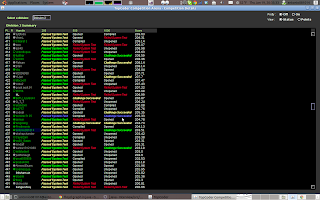## Thursday, January 19, 2012

### SRM530

Level 1 Level 2 Level 3
Div 1 Level 1 Level 2 Level 3
Div 2 Level 1 Level 2 Level 3

## Tutorials:SRM530

### Solution

The same with Div2-Lev2.

### Solution

Brute-force. Even here I made a minor mistake, which assume the cutter will start with a dot, what a joke.

### Source Code:

/**
* @author antonio081014
* @since Jan 19, 2012, 6:25:15 PM
*/

public class GogoXCake {
public String solve(String[] cake, String[] cutter) {
for (int i = 0; i + cutter.length <= cake.length; i++) {
for (int j = 0; j + cutter.length() <= cake[i].length(); j++) {
if (checkCutter(cake, cutter, i, j)) {
for (int x = 0; x < cutter.length; x++) {
for (int y = 0; y < cutter[x].length(); y++) {
if (cutter[x].charAt(y) == '.')
cake[i + x] = cake[i + x].substring(0, y + j)
+ "X"
+ cake[i + x].substring(y + j + 1);
}
}
}
}
}
return checkCake(cake) ? "YES" : "NO";
}

public void print(String[] cake) {
for (int i = 0; i < cake.length; i++)
System.out.println(cake[i]);
}

public boolean checkCake(String[] cake) {
for (int i = 0; i < cake.length; i++)
for (int j = 0; j < cake[i].length(); j++)
if (cake[i].charAt(j) == '.')
return false;
return true;
}

public boolean checkCutter(String[] cake, String[] cutter, int x, int y) {
for (int i = 0; i < cutter.length; i++) {
for (int j = 0; j < cutter[i].length(); j++) {
if (cutter[i].charAt(j) == '.')
if (cake[i + x].charAt(j + y) != cutter[i].charAt(j)) {
return false;
}
}
}
return true;
}

// <%:testing-code%>
}

### Solution

I used the brute-force, which go through all the permutations.

### Source Code:

//Thursday, January 19, 2012 17:51 PST
#include <vector>
#include <list>
#include <map>
#include <set>
#include <deque>
#include <stack>
#include <bitset>
#include <algorithm>
#include <functional>
#include <numeric>
#include <utility>
#include <sstream>
#include <iostream>
#include <iomanip>
#include <cstdio>
#include <cmath>
#include <cstdlib>
#include <ctime>

using namespace std;

class GogoXBallsAndBinsEasy {
public:
int solve(vector <int>);
};

int GogoXBallsAndBinsEasy::solve(vector <int> T) {
vector<int> v(T.begin(), T.end());
sort(v.begin(), v.end());
sort(T.begin(), T.end());
int count = 0;
do{
int tmp = 0;
for(int i=0; i<v.size(); i++){
tmp += (v[i] - T[i]) >0? (v[i]-T[i]) : 0;
}
count = max(count, tmp>0? tmp:-tmp);
}while(next_permutation(v.begin(), v.end()));
return count;
}